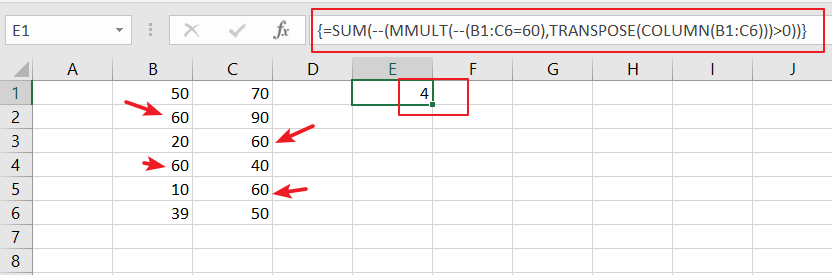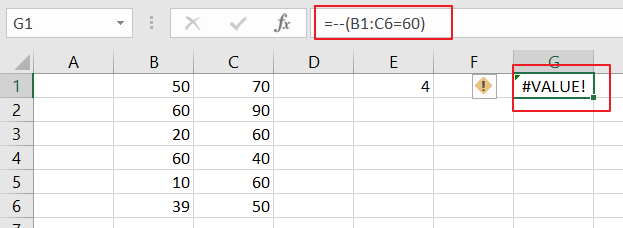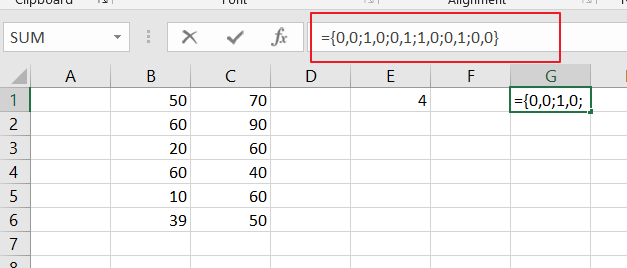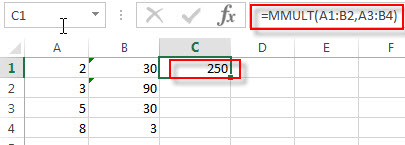## Excel Array Matrix Operation

In Excel, we have a built-in function used to perform matrix operations. It is the MMULT function. This function accepts two arrays as parameters and returns the product of the two arrays. However, this function has a prerequisite that the number of rows and columns of the two arrays passed in are the same.

MMULT function is used to calculate the matrix product of two arrays, its syntax structure is as below:

``=MMULT (arrayl, array2)``

where `array1`, `array2 `are the two arrays to be multiplied. the number of columns of `array1 `and the number of rows of `array2 `must be the same, and both arrays can only contain numeric elements.

## Excel Array Matrix Operation Example

If you have an interview score sheet, you need to score `Secretarial Skills`, `Interpersonal Skills`, and `Professional Behavior` and calculate the final score. The Secretarial Skills, Interpersonal Skills, and Professional Behavior scores are weighted at `0.3`, `0.5`, and `0.2`, respectively.

In order to calculate the final interview scores for each person, you need to perform a matrix product of the skill item scores and the weighted value scores by using the MMULT function, which you can refer to the following steps.

STEP 1# Select the cell area `G3:G11`

STEP2# In the formula bar, enter the following array formula

``=MMULT(D3:F11,I3:I5)``

STEP3# Press Ctrl+Shift+Enter shortcut keys to convert the above ordinary formula into an array formula.

``{=MMULT(D3:F11,I3:I5)}``

From the screenshot above, you can see that `TOM `had the best interview score, his interview score was `4.7`.

Since the interview results table on the left and the weight table on the right are mutually corresponding, you can use the MMULT function to calculate the matrix product of the array of scores and the array of weights to get the final interview results. The MMULT function returns an array of results, which must be entered as a multi-cell array formula in order to fill the `G3:G11` cell area with the results.

## How to Count Row That Contain Specific Value in Excel

This post will guide you how to count rows that contain a specific text or number in Excel 2013/2016 or Excel office 365. How do I count the number of rows that contain a particular value while working with your current worksheet in Excel. And you can do this by a array formula based on the SUM function, the MMULT function, the TRANSPOSE function and the COLUMN function in Excel.

## General Formula:

The below general formula can help you to count rows that have specific values in Excel. Like this:

`=SUM(--(MMULT(--(criteria), TRANSPOSE(COLUMN(Range)))>0))`

Note: this formula is an array formula and you have to press “`CTRL + SHITF +Enter`” to change it from a normal formula to a array formula.

## Count Row That Contain Specific Value

Assuming that you have a data of list in range B2:C6. And you want to know the number of rows that have a particular value 60 in your given range of cells.  And you can refer to the above generic formula to create a new array formula. Like this:

`=SUM(--(MMULT(--(B1:C6=60),TRANSPOSE(COLUMN(B1:C6)))>0))`Let’s See That How This Formula Works:

`=--(B2:C6=60)`This formula is a logic criteria, and it is used to generate a TRUE and FALSE array result, and the double negative operator can be used to force the TRUE and FALSE values to 1 and 0 respectively.

`{0,0;1,0;0,1;1,0;0,1;0,0}`The Column function can be used to get the column number in an array format. And the TRANSPOSE function is used to change the column array format to row array.

Finally, the SUM function will count all those rows that have your specific value in the given range of cells.

Related Functions

• Excel COLUMN function
The Excel COLUMN function returns the first column number of the given cell reference.The syntax of the COLUMN function is as below:=COLUMN ([reference])….
• Excel SUM function
The Excel SUM function will adds all numbers in a range of cells and returns the sum of these values. You can add individual values, cell references or ranges in excel.The syntax of the SUM function is as below:= SUM(number1,[number2],…)…
• Excel TRANSPOSE function
Excel TRANSPOSE formula allows you to rotate (swap) values from rows to columns and vice versa in Excel.The Excel TRANSPOSE Function syntax:=TRANSLATE (range) …

## Excel MMULT Function

This post will guide you how to use Excel MMULT function with syntax and examples in Microsoft excel.

### Description

The Excel MMULT function returns the matrix product of two arrays.

If you want to return multiple result in an array on the worksheet, then you need to input an array formula in the MMULT function.

To input array formulas, you need to highlight the range of cells that this function is to be entered into, and then type the function into the first cell of the range, and press shortcuts Ctrl + Shift + Enter.

And the returned array result will contain the same number of rows as array1 and the same number of columns as array2.

The MMULT function is a build-in function in Microsoft Excel and it is categorized as a Math and Trigonometry Function.

The MMULT function is available in Excel 2016, Excel 2013, Excel 2010, Excel 2007, Excel 2003, Excel XP, Excel 2000, Excel 2011 for Mac.

### Syntax

The syntax of the MMULT function is as below:

`=MMULT (array1,array2)`

Where the MMULT function arguments are:

• array1 – This is a required argument. The first array that you wish to multiply.
• array2 – This is a required argument. The second array that you wish to multiply.

Note:

• The array can be a cell range or an array constant, such as: {2,3;5,6} and both arrays must contain only numeric values.
• If any cells in array are empty or contain text string, then the MMULT function will return the #VALUE! Error.
• If the Array does not have the same number of rows and columns, then the MMULT function will return the #VALUE! Error.
• Formulas that return arrays must be entered as array formulas.

### Excel MMULT Function Examples

The below examples will show you how to use Excel MMULT Function to calculate the matrix product of two arrays.

1# to calculate the matrix product of two arrays A1:B2 and A3:B4, enter the following formula in Cell D1.

`=MMULT(A1:B2,A3:B4)`### Related Functions

• Excel MDETERM function
The Excel MDETERM function returns the matrix determinant of an array. So you can use the MDETERM function to calculate the determinant of a square matrix in Excel.The syntax of the MDETERM function is as below:=MDETERM(array)…
• Excel MINVERSE Function
The Excel MINVERSE function returns the inverse matrix for a given matrix.The syntax of the MINVERSE function is as below:=MINVERSE(array)…